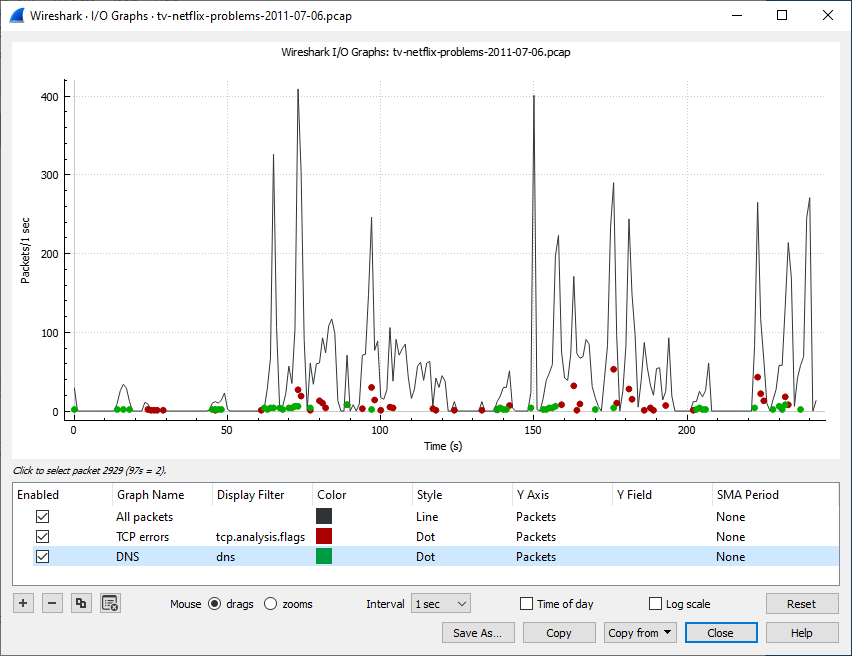## 8.8. The “I/O Graphs” Window

Lets you plot packet and protocol data in a variety of ways.

Figure 8.6. The “I/O Graphs” windowAs shown above, this window contains a chart drawing area along with a customizable list of graphs. Graphs are saved in your current profile. They are divided into time intervals, which can be set as described below. Hovering over the graph shows the last packet in each interval except as noted below. Clicking on the graph takes you to the associated packet in the packet list. Individual graphs can be configured using the following options:

Enabled
Draw or don’t draw this graph.
Graph Name
The name of this graph.
Display Filter
Limits the graph to packets that match this filter.
Color
The color to use for plotting the graph’s lines, bars, or points.
Style
How to visually represent the graph’s data, e.g. by drawing a line, bar, circle, plus, etc.
Y Axis

The value to use for the graph’s Y axis. Can be one of:

Packets, Bytes, or Bits
The total number of packets, packet bytes, or packet bits that match the graph’s display filter per interval. Zero values are omitted in some cases.
SUM(Y Field)
The sum of the values of the field specified in “Y Field” per interval.
COUNT FRAMES(Y Field)
The number of frames that contain the field specified in “Y Field” per interval. Unlike thie plain “Packets” graph, this always displays zero values.
COUNT FIELDS(Y Field)
The number of instances of the field specified in “Y Field” per interval. Some fields, such as dns.resp.name, can show up multiple times in a packet.
MAX(Y Field), MIN(Y Field), AVG(Y Field)
The maximum, minimum, and arithmetic mean values of the specified “Y Field” per interval. For MAX and MIN values, hovering and clicking the graph will show and take you to the packet with the MAX or MIN value in the interval instead of the most recent packet.

If the “Y Field” is a relative time value, this is the sum of the “Y Field” values divided by the interval time. This can be useful for tracking response times.

Y Field
The display filter field from which to extract values for the Y axis calculations listed above.
SMA Period
Show an average of values over a specified period of intervals.

The chart as a whole can be configured using the controls under the graph list:

Copy the selected graph.
Remove all graphs.
Mouse drags / zooms
When using the mouse inside the graph area, either drag the graph contents or select a zoom area.
Interval
Set the interval period for the graph.
Time of day
Switch between showing the absolute time of day or the time relative from the start of capture in the X axis.
Log scale
Switch between a logarithmic or linear Y axis.

The main dialog buttons along the bottom let you do the following:

The button will take you to this section of the User’s Guide.

The button will copy values from selected graphs to the clipboard in CSV (Comma Separated Values) format.

will let you copy graphs from another profile.

will close this dialog.

will save the currently displayed graph as an image or CSV data.

TipYou can see a list of useful keyboard shortcuts by right-clicking on the graph.

#### Missing Values Are Zero

Wireshark’s I/O Graph window doesn’t distinguish between missing and zero values. For scatter plots it is assumed that zero values indicate missing data, and those values are omitted. Zero values are shown in line graphs, and bar charts.